# NCERT Solutions for Class 10 Maths Exercise 1.4## myCBSEguide App

CBSE, NCERT, JEE Main, NEET-UG, NDA, Exam Papers, Question Bank, NCERT Solutions, Exemplars, Revision Notes, Free Videos, MCQ Tests & more.

NCERT solutions for Class 10 Maths Real Numbers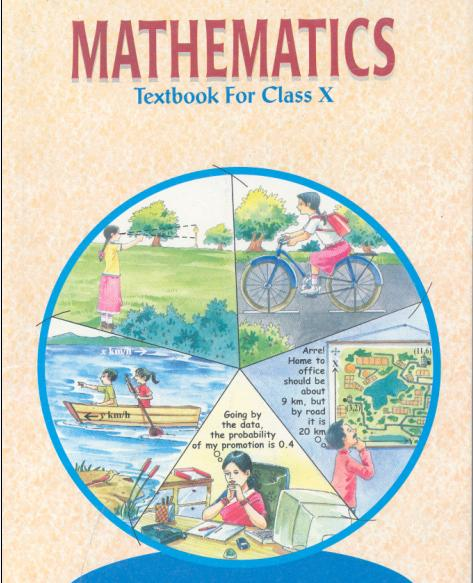## NCERT Solutions for Class 10 Maths Real Numbers

###### 1. Without actually performing the long division, state whether the following rational numbers will have a terminating decimal expansion or a non-terminating decimal expansion.

(i)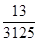(ii)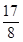(iii)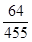(iv)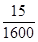(v)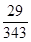(vi)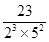(vii)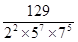(viii)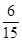(ix)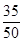(x)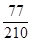###### Ans. According to Theorem, any given rational number of the form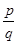where p and q are co-prime, has a terminating decimal expansion if q is of the form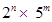, where m and n are non-negative integers.

(i)q = 3125 =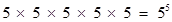Here, denominator is of the form, where m = 5 and n = 0.

It means rational numberhas a terminating decimal expansion.

(ii)q = 8 =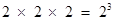Here, denominator is of the form, where m = 0 and n = 3.

It means rational numberhas a terminating decimal expansion.

(iii)q = 455 =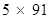Here, denominator is not of the, where m and n are non-negative integers.

It means rational numberhas a non-terminating repeating decimal expansion.

(iv)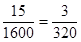q = 320 =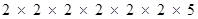=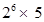Here, denominator is of the form, where m = 1 and n = 6.

It means rational numberhas a terminating decimal expansion.

(v)q = 343 =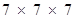Here, denominator is not of the form, where m and n are non-negative integers.

It means rational numberhas non-terminating repeating decimal expansion.

(vi)q =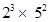Here, denominator is of the form, where m = 2 and n = 3 are non-negative integers.

It means rational numberhas terminating decimal expansion.

(vii)q =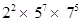Here, denominator is not of the form, where m and n are non-negative integers.

It means rational numberhas non-terminating repeating decimal expansion.

(viii)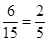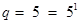Here, denominator is of the form, where m = 1 and n = 0.

It means rational numberhas terminating decimal expansion.

(ix)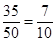q = 10 =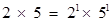Here, denominator is of the form, where m = 1 and n = 1.

It means rational numberhas terminating decimal expansion.

(x)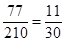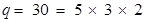Here, denominator is not of the form, where m and n are non-negative integers.

It means rational numberhas non-terminating repeating decimal expansion.

NCERT Solutions for Class 10 Maths Exercise 1.4

###### 2. Write down the decimal expansions of those rational numbers in Question 1 which have terminating decimal expansions.

Ans. (i)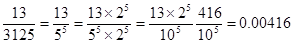(ii)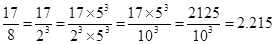(iv)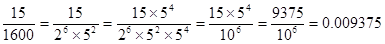(vi)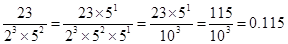(viii)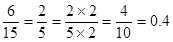(ix)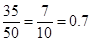NCERT Solutions for Class 10 Maths Exercise 1.4

###### 3. The following real numbers have decimal expansions as given below. In each case, decide whether they are rational or not. If, they are rational, and of the form, what can you say about the prime factors of q?

(i) 43.123456789

(ii) 0.1201120012000120000…

(iii)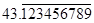Ans. (i) 43.123456789

It is rational because decimal expansion is terminating. Therefore, it can be expressed inform where factors of q are of the form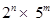where n and m are non-negative integers

(ii) 0.1201120012000120000…

It is irrational because decimal expansion is neither terminating nor non-terminating repeating.

(iii)It is rational because decimal expansion is non-terminating repeating. Therefore, it can be expressed inform where factors of q are not of the formwhere n and m are non-negative integers.

## NCERT Solutions for Class 10 Maths Exercise 1.4

NCERT Solutions Class 10 Maths PDF (Download) Free from myCBSEguide app and myCBSEguide website. Ncert solution class 10 Maths includes text book solutions from Mathematics Book. NCERT Solutions for CBSE Class 10 Maths have total 15 chapters. 10 Maths NCERT Solutions in PDF for free Download on our website. Ncert Maths class 10 solutions PDF and Maths ncert class 10 PDF solutions with latest modifications and as per the latest CBSE syllabus are only available in myCBSEguide.

## CBSE app for Class 10

To download NCERT Solutions for Class 10 Maths, Computer Science, Home Science,Hindi ,English, Social Science do check myCBSEguide app or website. myCBSEguide provides sample papers with solution, test papers for chapter-wise practice, NCERT solutions, NCERT Exemplar solutions, quick revision notes for ready reference, CBSE guess papers and CBSE important question papers. Sample Paper all are made available through the best app for CBSE students and myCBSEguide website.### 12 thoughts on “NCERT Solutions for Class 10 Maths Exercise 1.4”

1. It was helpful thank u very much

2. Very nice

3. Very nice

4. Tysm!

5. It helped me a lot…Thank you so much!

6. It is more different than which our sar teach

7. Very nice

8. Thanks ,my teacher doesn’t teach me well .it’s very helpful……..

9. Thank you so much…

10. Very nice

11. thankyou sir

12. Thanks a lot